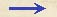Discorsi Propositions 2/01-th-01 Discorsi Proposition2/01-th-01THEOREMA I, PROPOSITIO I. THEOREM I, PROPOSITION ITempus in quo aliquod spatium a mobili conficitur latione ex quiete uniformiter accelerata, est aequale tempori in quo idem spatium {10} conficeretur ab eodem mobili motu aequabili delato, cuius velocitatis gradus subduplus sit ad summum et ultimum gradum velocitatis prioris motus uniformiter accelerati. The time in which any space is traversed by a body starting from rest and uniformly accelerated is equal to the time in which that same space would be traversed by the same body moving at a uniform speed whose value is the mean of the highest speed and the speed just before acceleration began. Repraesentetur per extensionem AB tempus in quo a mobili latione uniformiter accelerata ex quiete in C conficiatur spatium CD; graduum autem velocitatis adauctae in instantibus temporis ab maximus et ultimus repraesentetur per EB, utcunque super AB constitutam; iunctaque AE, lineae omnes ex singulis punctis lineae ab ipsi BE aequidistanter actae, crescentes velocitatis gradus post instans A repraesentabunt.{20} Divisa deinde BE bifariam in F, ductisque parallelis FG, AG ipsis BA, BF, parallelogrammum AGFB erit constitutum, triangulo AEB aequale, dividens suo latere GF bifariam AE in I: quodsi parallelae trianguli AEB usque ad IG extendantur, habebimus aggregatum parallelarum omnium in quadrilatero contentarum aequalem aggregatui comprehensarum in triangulo AEB; quae enim sunt in triangulo IEF, pares sunt cum contentis in triangulo GIA; eae vero quae habentur in trapezio AIFB, communes sunt. Cumque singulis et omnibus {30} instantibus temporis AB respondeant singula et omnia puncta lineae AB, ex quibus actae parallelae in triangulo AEB comprehensae crescentes gradus velocitatis adauctae repraesentant, parallelae vero intra parallelogrammum contentae totidem gradus velocitatis non adauctae, sed aequabilis, itidem {209} repraesentent; apparet, totidem velocitatis momenta absumpta esse in motu accelerato iuxta crescentes parallelas trianguli AEB, ac in motu aequabili iuxta parallelas parallelogrammi GB: quod enim momentorum deficit in prima motus accelerati medietate (deficiunt enim momenta per parallelas trianguli AGI repraesentata), reficitur a momentis per parallelas trianguli IEF repraesentatis. Patet igitur, aequalia futura esse spatia tempore eodem a duobus mobilibus peracta, quorum unum motu ex quiete uniformiter accelerato moveatur, alterum vero motu aequabili iuxta momentum subduplum momenti maximi velocitatis accelerati motus: quod erat intentum. Let us represent by the line AB the time in which the space CD is traversed by a body which starts from rest at C and is uniformly accelerated; let the final and highest value of the speed gained during the interval AB be represented by the line EB drawn at right angles to AB; draw the line AE, (Condition 2/00-th-00-dialog1) then all lines drawn from equidistant points on AB and parallel to BE will represent the increasing values of the speed, beginning with the instant A. Let the point F bisect the line EB; draw FG parallel to BA, and GA parallel to FB, thus forming a parallelogram AGFB which will be equal in area to the triangle AEB, since the side GF bisects the side AE at the point I; for if the parallel lines in the triangle AEB are extended to GI, then the sum of all the parallels contained in the quadrilateral is equal to the sum of those contained in the triangle AEB; for those in the triangle IEF are equal to those contained in the triangle GIA, while those included in the trapezium AIFB are common.Since each and every instant of time; in the time-interval AB has its corresponding point on the line AB, from which points parallels drawn in and limited by the triangle AEB represent to increasing values of the growing velocity, and since parallels contained within the rectangle represent the values of a speed which is not increasing, but constant, it appears, in like manner, that the momenta (momenta) assumed by the moving body may also be represented, in the case of the accelerated motion, by the increasing parallels of the triangle AEB, and, in the case of the uniform motion, by the parallels of the rectangle GB. For, what the momenta may lack in the first part of the accelerated motion (the deficiency of the momenta being represented by the parallels of the triangle AGI) is made up by the momenta represented by the parallels of the triangle IEF. (Condition Aristot-space-prop) Hence it is clear that equal spaces will be traversed in equal times by two bodies, one of which, starting from rest, moves with uniform acceleration, while the momentum of the other, moving with uniform speed, is one-half its maximum momentum under accelerated motion. Q. E. D.Discorsi Propositions 2/01-th-01 Discorsi Proposition2/01-th-01